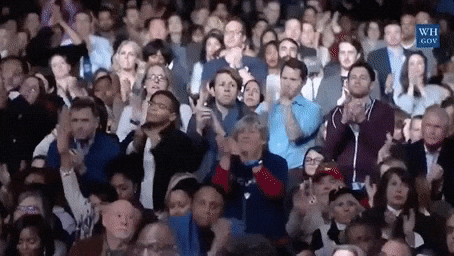Press 'Use this template' button at the top-right corner to copy this template and you can edit and use it.
Math / English Quiz

Math

Solve the entire problem set, and we will provide you with feedback including an error log after grading.

1 + 1 = ?

1

2

3

4

3 * 2 = ?

4

5

6

7

2 * ( 4 - 2 ) = ?

6

1

3

4

2 + 4 * 3 = ?

18

24

14

16

English

Solve the entire problem set, and we will provide you with feedback including an error log after grading.

I ______ a boy.

am

are

is

will

You _____ a girl.

am

are

is

will

A: How are you?
B: ___________________

B: No, I am not.

B: Yes, you can.

B: I will do it.

B: Fine, thank you, and you?

A: Thank you.
B: __________________.

B: You can do it.

B: You're welcome.

B: Yes, I can.

B: Good morning.

Your total score is/ 200. (Correct answer rate:%)

Math score:/ 100.

English score:/ 100.Lacking a lot!Need more effort!Very good!Excellent!

All Math is correct! Excellent!

You gotout of 4 questions wrong on Math.

1 + 1 = 2
You have answered.

3 * 2 = 6
You have answered.

2 * ( 4 - 2 ) = 2
You have answered.
If there are parentheses in a formula, always evaluate inside the parentheses first.

2 + 4 * 3 = 14
You have answered.
If addition, subtraction, multiplication, and division are mixed in the formula without parentheses, multiplication and division must be calculated first.

All English is correct. Excellent!

You gotout of 4 questions wrong on English.

I am a boy.
You have answered.
It is correct to use 'am', the present tense of the verb 'be' in the first person.

You are a girl.
You have answered.
It is correct to use 'are', the present tense of the verb 'be' in the second person.

A: How are you?
B: Fine Thank you, and you?
You have answered.
It is an expression that is used idiomatically.

A: Thank you.
B: You're welcome.
You have answered.
This is an idiomatically used expression.